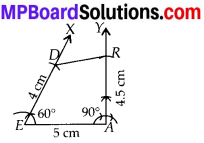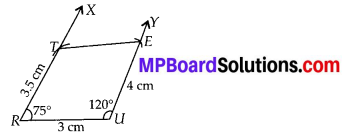# MP Board Class 8th Maths Solutions Chapter 4 Practical Geometry Ex 4.4

## MP Board Class 8th Maths Solutions Chapter 4 Practical Geometry Ex 4.4

Question 1.
DEAR
DE = 4 cm
EA = 5 cm
AR = 4.5 cm
∠E = 60°
∠A = 90°TRUE
TR = 3.5 cm
RU = 3 cm
UE = 4 cm
∠R = 75°
∠U = 120°
Solution:
(i) Steps of Construction:
Step-1: Draw EA = 5 cm.
Step-2: Make ∠AEX = 60° and ∠EAY = 90°.
Step-3 : Cut off arcs AR = 4.5 cm on $$\overrightarrow{A Y}$$ and ED = 4 cm on $$\overrightarrow{E X}$$.
Step-4: Join DR.
Hence, DEAR is the required quadrilateral.(ii) Steps of Construction :
Step-1: Draw RU = 3 cm.
Step-2 : Make ∠URX = 75° and ∠RUY = 120°
Step-3 : Cut off RT = 3.5 cm on $$\overrightarrow{R X}$$ and UE = 4 cm on $$\overrightarrow{U Y}$$.
Step-4: Join TE.
Hence, TRUE is the required quadrilateral.